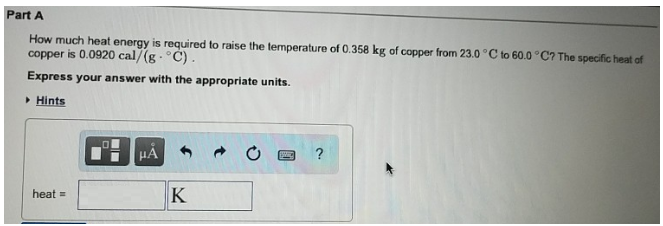Chemistry Calorimetry Solution: How much heat energy is required to raise the temp...

🤓 Based on our data, we think this question is relevant for Professor Banks' class at NCSU.

# Solution: How much heat energy is required to raise the temperature of 0.358 kg of copper from 23.0°C to 60.0°C? The specific heat of copper is 0.0920 cal/(g • °C). Express your answer with the appropriate units.

Problem

How much heat energy is required to raise the temperature of 0.358 kg of copper from 23.0°C to 60.0°C? The specific heat of copper is 0.0920 cal/(g • °C). Express your answer with the appropriate units.View Complete Written Solution